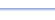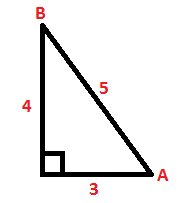Program: SOHCAHTO

SOHCAHTO

• Displays "SOH CAH TOA" on the screen.

Useful for:

• ACT
• Geometry
• Precalculus
• Trigonometry
• Calculus

See full list of programs

Example

Q: Find sin A, cos A, and tan A.

Solution:

Run SOHCAHTO.  How?

The program displays:

This reminds you that:
sin x = Opposite / Hypotenuse
cos x = Adjacent / Hypotenuse